Ex 11.2

Chapter 11 Class 9 Constructions [Deleted]
Serial order wise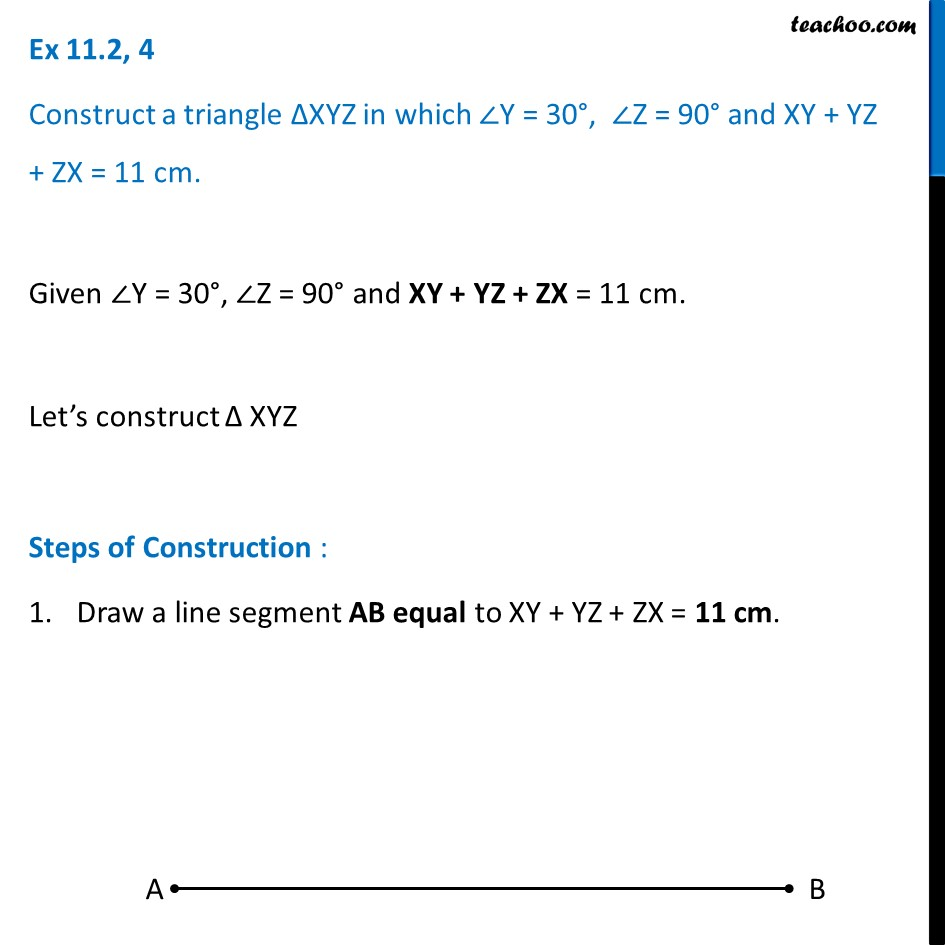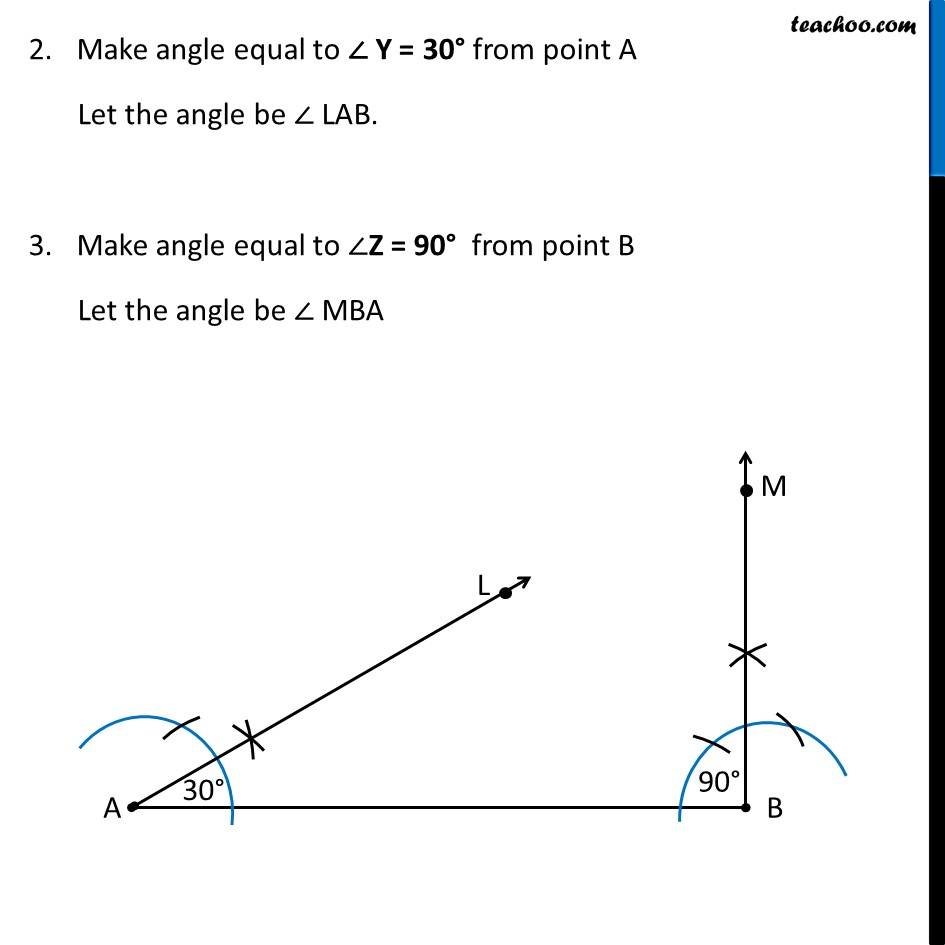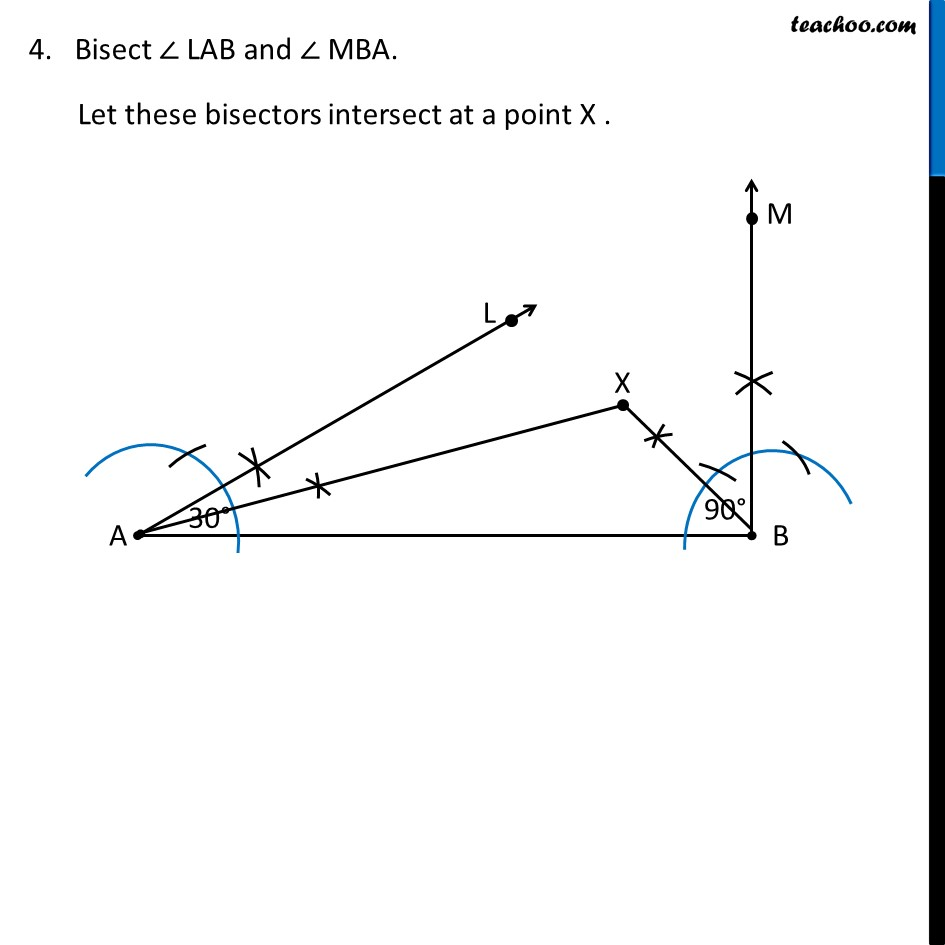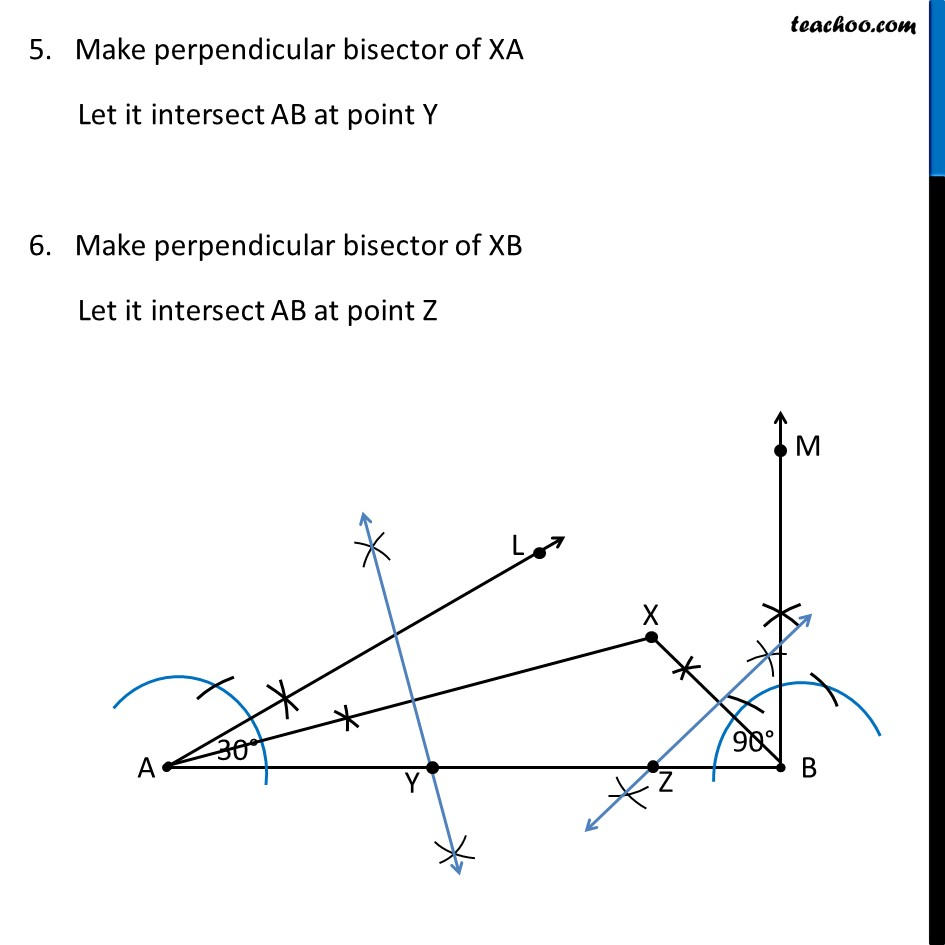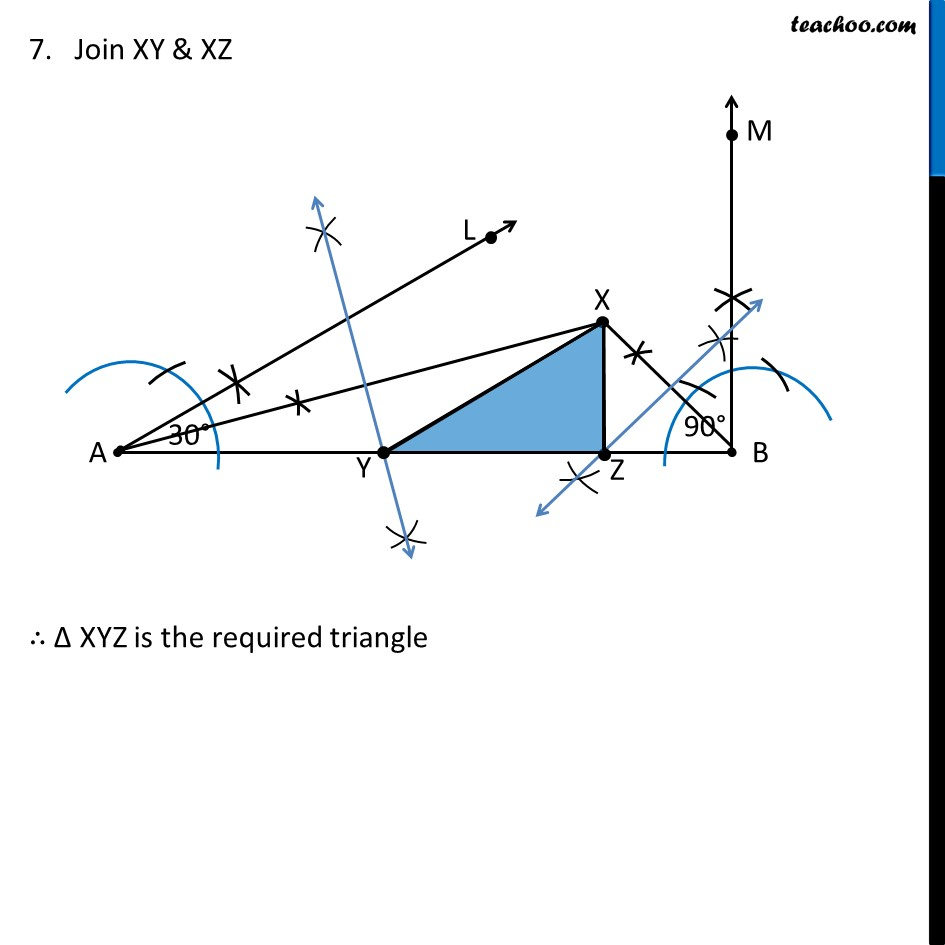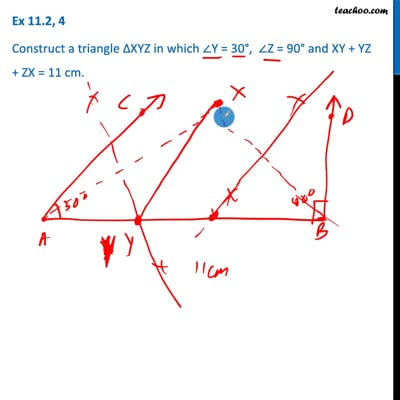This video is only available for Teachoo black users

Maths Crash Course - Live lectures + all videos + Real time Doubt solving!

### Transcript

Ex 11.2, 4 Construct a triangle ∆XYZ in which ∠Y = 30°, ∠Z = 90° and XY + YZ + ZX = 11 cm. Given ∠Y = 30°, ∠Z = 90° and XY + YZ + ZX = 11 cm. Let’s construct Δ XYZ Steps of Construction : Draw a line segment AB equal to XY + YZ + ZX = 11 cm. Make angle equal to ∠ Y = 30° from point A Let the angle be ∠ LAB. Make angle equal to ∠Z = 90° from point B Let the angle be ∠ MBA 4. Bisect ∠ LAB and ∠ MBA. Let these bisectors intersect at a point X . 5. Make perpendicular bisector of XA Let it intersect AB at point Y 6. Make perpendicular bisector of XB Let it intersect AB at point Z 7. Join XY & XZ ∴ Δ XYZ is the required triangle